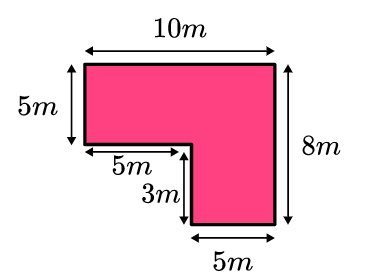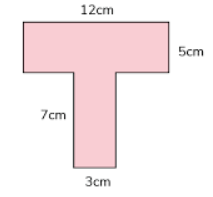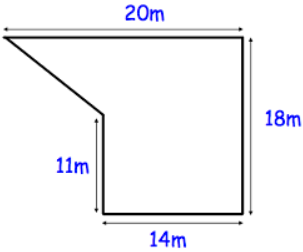# Geometric Measurements Of Different Shapes

This quiz covers the topic of geometric measurements of different shapes like triangles, rectangles, circles, and so on. This quiz contains multiple-choice questions as well as some fill-in-the-blanks too.

Start Quiz

If the area of a triangle is 15 cm squares, and the base is 2 cm, then what is the height?

20 cm

15 cm

5 cm

30 cm

If the area of a triangle is 20 cm squares, and the base is 4 cm, then what is the height?

20 cm

15 cm

5 cm

10 cm

If the length and breadth of a rectangle are 12m and 15m respectively. Find the perimeter of the rectangle.

12 meters

54 meters

27 meters

108 meters

If the length and breadth of a rectangle are 4m and 5m respectively. Find the area of the rectangle.

10 meter squares

40 meter squares

20 meter squares

25 meter squares

A rectangular schoolyard is 150 meters long and 200 meters wide. Find the perimeter of the schoolyard.

Perimeter= ##700## meters

700

The radius of a circle is 2 meters. What is the area of the circle?

(Use pie= 3.14)

Area= ##12.56## meter squares

12.56

The radius of a circle is 5cm. What is the circle’s circumference?

(Use pie= 3.14)

Area= ##31.4## centimeters

31.4

Find the area of the given figure.Area= ##65## meter squares

65

Find the area of the given figure.Area= ##81## centimeter squares

81

Find the area of the given figure.Area= ##273## meter squares

273
Quiz/Test Summary
Title: Geometric Measurements Of Different Shapes
Questions: 10
Contributed by: